Q.1) How many minutes is it past the hour?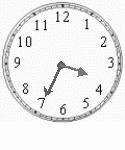a)  45 minutes
b)  35 minutes
c)  25 minutes

Q.2) What time is it?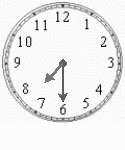a)  7:30
b)  6:30
c)  8:30

Q.3) Convert:
3 hours = ___ minutes
a) 180
b) 120

Q.4) Convert:
8 days = ___ hours
a) 192
b) 216

Q.5) Convert:
20 minutes = ___ seconds
a) 1260
b) 1200

Q.6) Convert 1917 hours in terms of 12 hours clock time?
a) 06:17 p.m.
b) 06:17 a.m.
c) 07:17 p.m.
d) 07:17 a.m.

Q.7) Convert 11:20 a.m. in terms of 24-hour clock time?
a) 0930 hours
b) 2320 hours
c) 1120 hours

Q.8) What time is 1 hours before 09:20 p.m.
a) 08:20 a.m.
b) 09:20 p.m.
c) 08:20 p.m.

Q.9) Add 4 minutes 40 seconds and 7 minutes 45 seconds.
a) 14 minutes 35 seconds
b) 12 minutes 25 seconds
c) 13 minutes 30 seconds

Q.10) Subtract 4 hours 30 minutes from 7 hours 15 minutes.
a) 3 hours 30 minutes
b) 2 hours 35 minutes
c) 2 hours 45 minutes

### Time Worksheets Grade 4 Explanations

Q.1) Explanation –

Minutes past the hour mean how many minutes have passed after completion of an hour.
i.e. here 35 minutes have passed after 3:00 so we can say it is 35 minutes past the hour.

Correct Answer – b)  35 minutes

Q.2) Explanation –

The hour hand is on between 7 and 8 so we will take it 7 and the minute hand is at 6 so minutes will be 6 × 5 = 30.

So, time = 7:30

Q.3) Explanation – Time Worksheets Grade 4

As we know that,
1 hours = 60 minutes
To find 3 hours in minutes. We have to multiply both sides by 3
( 3 x 1 ) hours = ( 3 x 60 ) minutes
3 hours = 180 minutes

Q.4) Explanation –

As we know that,
1 days = 24 hours
To find 8 days in hours. We have to multiply both sides by 8
( 8 x 1 ) days = ( 8 x 24 ) hours
8 days = 192 hours

Q.5) Explanation –

As we know that,
1 minutes = 60 seconds
To find 20 minutes in seconds. We have to multiply both sides by 20
( 20 x 1 ) minutes = ( 20 x 60 ) seconds
20 minutes = 1200 seconds

Q.6) Explanation – Time Worksheets Grade 4

1917 hours shows that the time is after noon.
In the above time, the first two digits represent hours and last two digits represents minutes.
We would first separate the hours digits (19), from minutes digits (17) by inserting a colon (:) between them
Hence, 1917 hours can be written as 19:17
Since we need to write the time in terms of 12 hours clock time, we would convert 19 hours into 12 hour time, by subtracting 12 from 19, i.e, 19-12 = 7
Now we would replace the 19 hours by 7 and since the time is after noon, we would add p.m. to the above time,
i.e, 07:17 p.m.
Take the difference between the number and 12, and replace hours by p.m.
Hence,1917 hours represent 07:17 p.m.

Correct Answer – c) 07:17 p.m.

Q.7) Explanation – Time Worksheets Grade 4

24-hours clock time does not contain a.m. or p.m., after the time. The time begins from midnight which is represent as 0000 hours. The time in 24-hours clock is represented by 4 digits, where, the first two digits represent hours and last two digits represents minutes
After 12 noon we keep counting as 13, 14…… rather than starting with 1 again.
So, to convert time into 24-hour clock if the given time is am then it will be the same in 24-hour clock
If the time is pm, we will add 12 to the given time to convert into 24-hour clock time.
Since, the time is 11:20 a.m., it would be written as 1120 hours in the 24-hours clock time.

Correct Answer – c) 1120 hours

Q.8) Explanation – Time Worksheets Grade 4

To find 1 hours before 09:20 p.m., we would need to add 1 hours to 09:20 p.m.
The hours would be 9 – 1 = 8
Since it is more than 12, the p.m. would continue as pm
Hence the time would be 8:20 pm.

Correct Answer – c) 08:20 p.m.

Q.9) Explanation – Time Worksheets Grade 4

Arrange minutes and seconds in columns, we get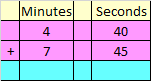40 seconds + 45 seconds = 85 seconds

= 60 seconds + 25 seconds

= 1 minute + 25 seconds

Write 25 below seconds column and carry over 1 to the minutes column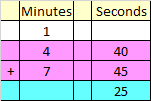Add the minutes and the carry over of 1 minute

4 minutes + 7 minutes + 1 minutes = 12 minutes

Write 12 under the minutes column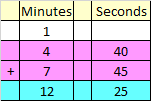Hence, 4 minutes 40 seconds + 7 minutes 45 seconds = 12 minutes 25 seconds

Correct Answer – b) 12 minutes 25 seconds

Q.10) Explanation – Time Worksheets Grade 4

Arranging hours and minutes in columns, we get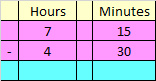Subtracting minutes : As 30 > 15 we would borrow 1 minute from 7 hours leaving 6 in the hours column.

After taking 1 as carry minutes would be

1 hour + 15 minutes = 75 minutes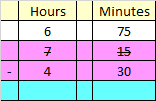Now subtract 30 minutes from 75 minutes , i.e,

75 minutes – 30 minutes = 45 minutes

Write 45 under minutes column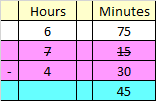Now subtract hours :-

6 hours – 4 hours = 2 hours

Write 2 under hours column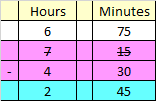Hence, 7 hours 15 minutes – 4 hours 30 minutes = 2 hours 45 minutes

Correct Answer – c) 2 hours 45 minutes